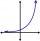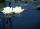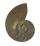Exp

If, then n is:

Result

n =  10

Solution:Leave us a comment of this math problem and its solution (i.e. if it is still somewhat unclear...):Be the first to comment!To solve this verbal math problem are needed these knowledge from mathematics:

Do you have a linear equation or system of equations and looking for its solution? Or do you have quadratic equation?

Next similar math problems:

1. Exponential equationFind x, if 625 ^ x = 5 The equation is exponential because the unknown is in the exponential power of 625
2. Theorem proveWe want to prove the sentence: If the natural number n is divisible by six, then n is divisible by three. From what assumption we started?
3. ProductThe product of two consecutive odd numbers is 8463. What are this numbers?
4. CalculationHow much is sum of square root of six and the square root of 225?
5. PowerNumber ?. Find the value of x.
6. Powers 32 to the power of n divided by 4 to the power of -3 equal 4. What is the vaule of n?
7. Exponential equationSolve for x: (4^x):0,5=2/64.
8. Water liliesWater lilies are growing on the pond and their number is doubled every day. The whole layer is covered in 12 days. How many days will it cover 8 layers?
9. EquationHow many real roots has equation ? ?
10. One halfOne half of ? is: ?
11. Intercept with axisF(x)=log(x+4)-2, what is the x intercept
12. Exponential equationSolve exponential equation (in real numbers): 98x-2=9
13. Logarithmic equationSolve equation: log33(3x + 21) = 0
14. Exponential equationIn the set R solve the equation: ?55%+36%+88%+71%+100=63% what is whole (X)? Percents can be added directly together if they are taken from the same whole, which means they have the same base amount. .. . You would add the two percentages to find the total amount.Between numbers 5 and 640 insert as many numbers to form geometric progression so sum of the numbers you entered will be 630. How many numbers you must insert?The group has 42 children. There are 4 more boys than girls. How many boys and girls are in the group?